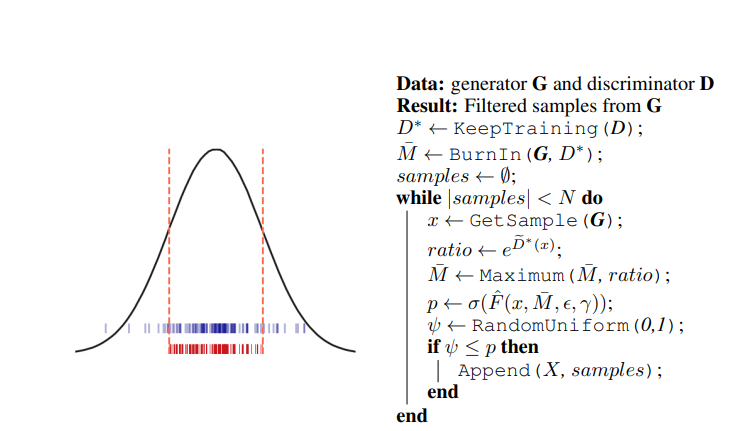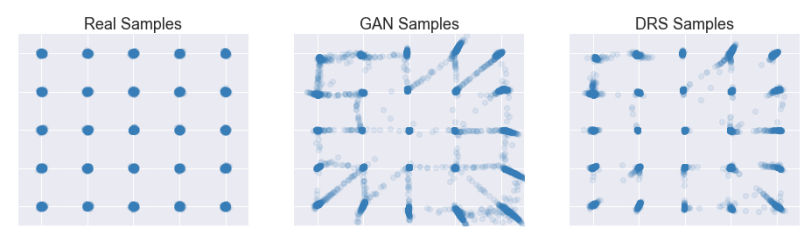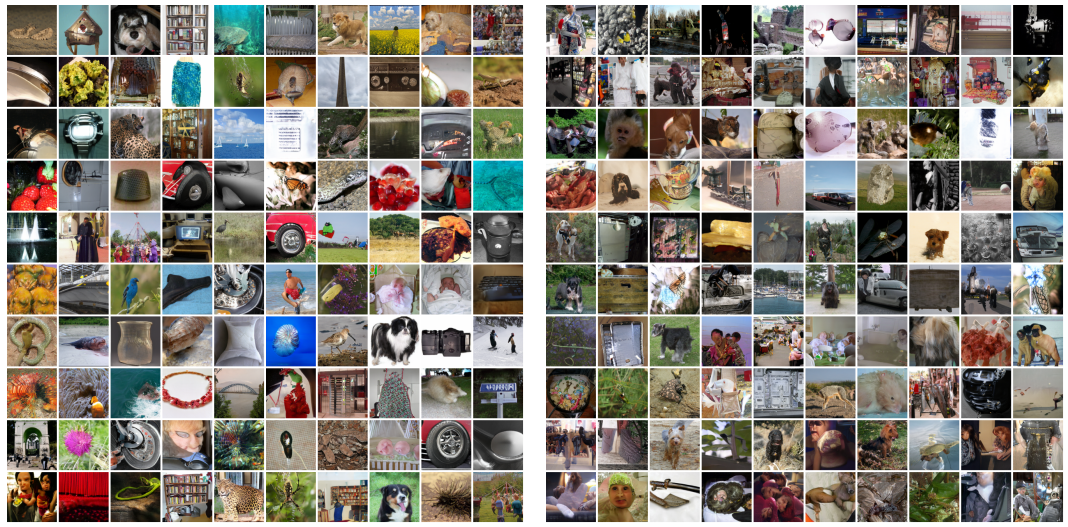Written by Michael (Mike) Erlihson, Ph.D.

This review is part of a series of reviews in Machine & Deep Learning that are originally published in Hebrew, aiming to make it accessible in a plain language under the name #DeepNightLearners.

Good night friends, today we are again in our section DeepNightLearners with a review of a Deep Learning article. Today I've chosen to review the article Rethinking Attention with Performers

### Reviewer Corner:

Reading recommendation From Mike: A must for GAN lovers, quite recommended for everyone else ;)
Clarity of writing: Medium-plus
Math and Deep Learning knowledge level: Good understanding in GAN training, basic knowledge in sampling methods, such as Rejection Sampling.
Practical applications: Improve generated image quality with GANs

### Article Details:

Published on: 26/02/2019 on Arxiv
Presented at: ICLR 2019

### Article Domains:

• Sample generation methods using trained GANs research

### Mathematical Tools, Concepts, and Marks:

• GANs
• Rejection Sampling

### The Article in Essence:

The article offers a method to improve the quality of generated images by a trained GAN while taking advantage of the accumulated "information" in the Discriminator (D) during the GAN training process. D is trained to distinguish between the generated images by the generator G to the original ones from the training set. D outputs the probability that the input image is a real one (from the training set). The article suggests using the image distribution, non implicitly held by D, to fix the image distribution held in G (the generated images), hence improving the generated image quality.

### The Basic Idea:

When D is trained well enough, it holds such a distribution over the image space that carries these attributes:

• Images that are similar to real photos receive a higher probability scores.
• Weird or unreal generated photos get lower probability scores.

It's more logical to sample from D's distribution because then there's a higher probability that we receive 'real-like images. But how can one sample from this distribution, when it is not intractable?

To overcome this difficulty, the authors are using samples from G with a sampling technique called Rejection Sampling, to sample from the D distribution.Figure 1: Left: For a uniform proposal distribution and Gaussian target distribution, the blue points are the result of rejection sampling and the red points are the result of naively throwing out samples for which the density ratio (pd(x)/pg(x)) is below a threshold. The naive method underrepresents the density of the tails. Right: the DRS algorithm. KeepTraining continues training using early stopping on the validation set. BurnIn computes a large number of density ratios to estimate their maximum. De∗ is the logit of D∗ . Fˆ is as in Equation 8. M¯ is an empirical estimate of the true maximum M.

### The article in brief:

When examining this idea deeper, one may question its efficiency. In theory, any valuable information contained in D's weights should have been transferred to G during the training. After all, the whole idea of GAN is based on transporting information from D to G through the GAN loss function gradient. However, there are several reasons why not all the accumulated data in D is transported to G.

1. These GAN training assumptions don't always happen. For example, both D and G have a limited capacity, and it's not always possible to transmit all the information from one to another through the network weights.
2. There may be situations when it is easier for D to distinguish between right and wrong distributions based on the samples rather than modeling correct distribution in D.
3. The most simplistic reason: the GAN may not be trained enough time for G to model its genuine distribution. The training process has ended before all the information is transformed from D to G.

Let's begin with a short explanation about rejection sampling, which is the core of the article idea.

### Rejection Sampling (RS)

RS is a technique to sample from a probability distribution, Pd, where direct sampling is hard or even impossible (e.g., when the distribution is implicit). Instead, we sample from another distribution, Pg, which is defined on the same space. But we can only sample from Pg if there is some constant M, which bounds the maximal ratio between the values of Pd and Pg. Here how it works:

We sample a point y from Pg, and we calculate the value of Pd at that y point. This Pd(y) is divided by M multiplied by Pg(y): t=Pd(y) / M*Pg(y). Sample y is accepted with the probability t and rejected with the probability 1-t.

We mark as Pd and Pg the distributions of D and G accordingly. But how can we perform RS if we can't explicitly calculate neither Pg nor Pd? The trick is that we only need to calculate the ratio, not the values that lead to it. The article states that under specific conditions ("ideal conditions") on Pd and Pg, one can accurately sample Pd through Pg.

### ideal conditions

1. Pd and Pg have the same supporting set - both are not equal to 0 at the same points.
2. The constant M (the maximum ratio between Pd and Pg) is known or calculable.
3. For a given generator G a discriminator D can be trained so that the GAN loss value is minimized to the absolute theoretical minima (this value equals LOG(4)). It is, of course, impossible since the sizes of the datasets and the length of the training are finite.

Under these conditions, the article shows that one can sample from Pd through Pg using RS. The formula for the ratio of Pd and Pg, in this case, includes the logit exponent of the optimal discriminator D*. This optimal D* is defined as such for which the loss function reaches the absolute minima. The proof is quite elegant and uses the formula for the optimal value of D* at the point x which equals the ratio `Pd(x) / ( Pd(x)+Pg(x) )` for G, marked as D*(x).

Of course, non of these conditions exist in real. The article suggests a method to perform RS despite the nonexistence of these ideal conditions.

For conditions 1) and 3), the article argues that a well-trained D is a good approximation of D*. When D is trained in such a way that prevents it from overfitting - using regularization, early stopping, etc., it can distinguish between good and bad samples, even if these samples have a probability 0 for the optimal Pd (for D*). They even empirically prove this assumption.

Regarding assumption 2) they suggest revaluing the constant M in two steps: a revaluation step where they calculate M's value over the first 10K samples (for each sample, M is the exponent of D's logit). Afterward, at the sampling step, M's value is updated to be the highest M's value of all the samples. It may lead to an over-revaluation of the calculated probabilities before updating M, but according to the article, updating M isn't too frequent.

Furthermore, the article states that RS has some difficulties when probing from high dimensional spaces since the probability t of getting a sample is very low. The authors suggest a nice "trick" to overcome this problem (which naturally distorts the actual sampling probability distribution): Introducing an additional parameter q, which extends the value set of the distribution. If the q parameter value is high, t tends to get relatively higher values too, and when the value of the q parameter is low, the value of t is low as well, and most of the samples are rejected. The q parameter value is determined through optimization.

## Achievements

The authors have improved the quality of the GAN-generated images with their method. They compared their images to SAGAN (trained on Imagenet), the SOTA of image generation, about two years ago.Real samples from 25 2D-Gaussian Distributions (left) as well as fake samples generated from a trained GAN model without (middle) and with DRS (right). Results are computed as an average over five models randomly initialized and trained independently.Synthesized images with the highest (left) and lowest (right) acceptance probability scores.

### Comparison metrics

Frechet Inception Distance

## P.S.

It is a paper with a brilliant idea behind it. Despite the impressive results, rigorous proofs of the authors' assumptions are missing, and I hope it will be introduced in the future.

#deepnightlearners

This post was written by Michael (Mike) Erlihson, Ph.D.

Michael works in the cybersecurity company Salt Security as a principal data scientist. Michael researches and works in the deep learning field while lecturing and making scientific material more accessible to the public audience.